# Test: Instruction Sets & Data Formats

## 10 Questions MCQ Test Topicwise Question Bank for Electronics Engineering | Test: Instruction Sets & Data Formats

Description
Attempt Test: Instruction Sets & Data Formats | 10 questions in 30 minutes | Mock test for Electronics and Communication Engineering (ECE) preparation | Free important questions MCQ to study Topicwise Question Bank for Electronics Engineering for Electronics and Communication Engineering (ECE) Exam | Download free PDF with solutions
QUESTION: 1

### A microprocessor using a 3 MHz clock has three T ' states in each machine cycle. What is the time taken to complete the execution of an instruction if the instruction cycle of this instruction needs 4 machine cycles?

Solution:

Number of T-states in each machine cycle = 3 So, number of T-states in 4 machine cycles = 3 x 4 = 12

Given, f = 3 MHz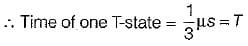Thus, time required for execution of the instruction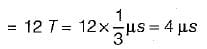QUESTION: 2

### Which of the following instruction will alter the contents of accumulator?

Solution:
• Instruction ‘CMP C’ compares the contents of register C with the content of accumulator A but contents of A and C will remain unchanged.
• Instruction ‘CPI 3A H' compares the 8-bit data 3A H with the contents of accumulator A but contents of A and C will remain unchanged.
• In instruction ‘AN! 5C H’, the 8-bit data 5C H is ANDed with contents of A and result is stored in A. So, the content of accumulator changes.
• In instruction ‘ORA A’, contents of A are ORed with contents of A itself and result is stored in A. But, the result of operation remains same as contents before execution ' of ORA A.

Hence, the contents of A does not change.

QUESTION: 3

### The addressing mode in which the machine language instruction itself includes the data is called

Solution:

In immediate addressing mode, an 8 bit or 16 bit data Is given In the instruction as operand.

QUESTION: 4

In an 8085 microprocessor, the accumulator contents are AAFI. After the execution of the instruction ‘CPI 99PT in the microprocessor

Solution:

Initially contents of accumulator A = AA H.
The instruction CPI 99 H compares the data 99H w ith the content of accumulator (A). This comparison is made by subtracting 99 H from contents of A i.e. AA H.
The contents of accumulator will remain unaffected but status of result will be reflected by flags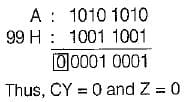Hence, after comparison both carry and zero flags will be reset

QUESTION: 5

The content of TEMP location after the execution of the following program in a 8085 microprocessor is:
MVI B, 00H
MVI A, IC H
DCR B
DAA
STA TEMP
HLT

Solution: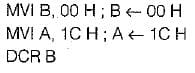Decrement the content of B by one (initially B = 00 H)

B= FF H

D A A ; D A A instruction changes the binary values of contents of accumulator to BCD.
Since lower order nibble of A is more than 9 (i.e. C), AC flag is set and upper nibble is less than 9, therefore DAA adds 0110 H to lower order nibble of A to adjust binary result to Binary coded decimal.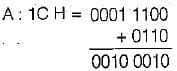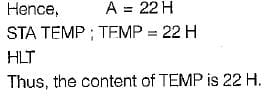QUESTION: 6

The following program is executed in the 8085 microprocessor: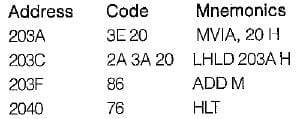What are the contents of the accumulator after the execution of above program?

Solution: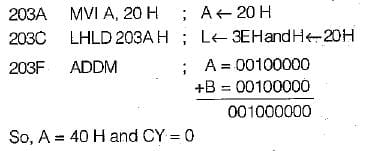2040 HLT ; Halts the execution of program Hence, the content of accumulator after the execution of given program are 40 H.

QUESTION: 7

What is the addressing mode used in the instruction PUSH D

Solution:

PUSH D stores the content of register pair DE on two top locations of stack, it is a 1 byte instruction and uses register indirect addressing mode.

QUESTION: 8

What is the effect of a DAD H instruction? ​

Solution:
QUESTION: 9

An instruction cycle is the time in which, a hardwired controlier completes four functions. The correct sequence of these functions is

Solution:

An instruction cycle is the time in which, a hardwired controller completes the following four functions;
(i) fetching of code from memory
(ii) updating the program counter
(iii) decoding of the instruction code
(iv) execution of the instruction

QUESTION: 10

Which of the following instruction does not use the stack?

Solution:

CALL and RET instruction uses stack while JMP instruction does not uses stack.Use Code STAYHOME200 and get INR 200 additional OFF Use Coupon Code